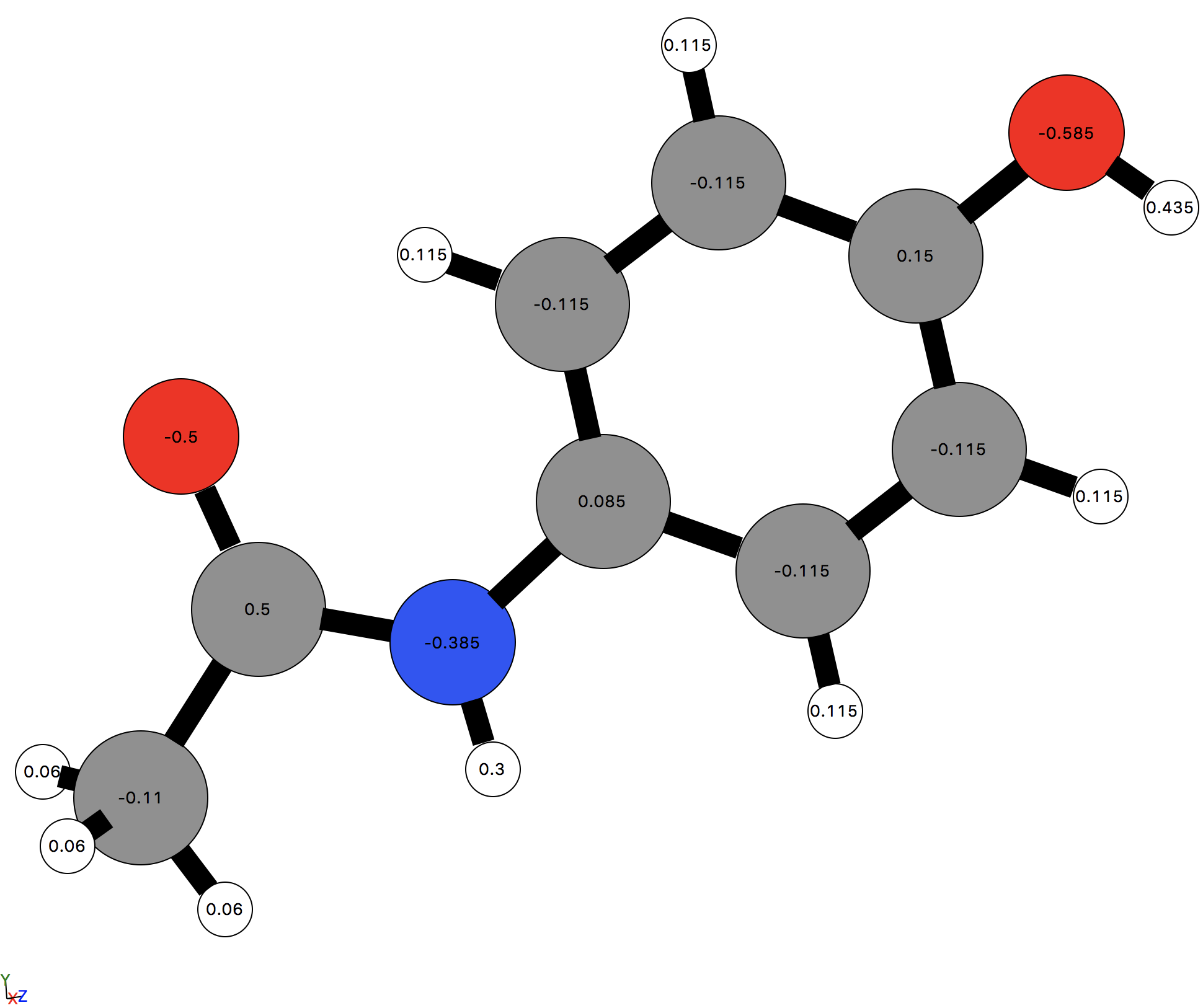# Reading atoms from configuration files

MDMC enables reading atomic configurations and topologies (if included) from configuration files.

The implemented file formats are:

In theory, it is possible to read a configuration file by simply passing it to the read function, as MDMC will determine the file format from the extension, if it is a supported format:

[ ]:

from MDMC.readers.configurations import read

# The return type of read is a list of atoms
print('The return type is: {}'.format(type(atoms)))

[ ]:

# We can use this to create a Molecule
from MDMC.MD import Molecule
paracetamol = Molecule(atoms=atoms)


The paracetamol Molecule read from the CIF file can be visualized using the view function:

[ ]:

from MDMC.gui import view
view(paracetamol)


From this it appears that the configuration and topology has been correctly read; however, MDMC does not automatically determine if certain atoms or bonded interactions are equivalent. For example, all three hydrogens in the methyl group are equivalent, as are the bonds, bond angles and dihedrals these hydrogens are involved in. In the case of paracetamol, there are 20 bonds in total, however only 12 of them are unique.

[ ]:

# To see all of the Bond interactions, we can filter the paracetamol interactions by name
bonds = list(filter(lambda x: x.name == 'Bond', paracetamol.interactions))

# There are 20 bonds in total
print('Number of bonds: {}'.format(len(bonds)))

# We can cast the list of bonds to a set to see the number of unique bonds - in this case it is still 20
unique_bonds = set(bonds)
print('Number of unique bonds: {}'.format(len(unique_bonds)))


There are two methods for specifying which atoms are equivalent; these can be seen by viewing the documentation relevant to the file format of the configuartion file, which can be accessed by passing docstring=True when calling read. For example, with a CIF file:

[ ]:

read('Paracetamol.cif', docstring=True)


So it is possible to define equivalent atoms either by specifying the name of each Atom or the atom_type of each Atom. These names or atom types must be passed in the same order as the atoms are defined within the CIF file (i.e. in the _atom_site_label identifier).

## Equivalence specified by atom_types

As with all atom_types specified in MDMC the integers used should be monotonically increasing.

[ ]:

# from MDMC.readers.configurations import read
atoms = read('data/Paracetamol.cif', atom_types=[1, 2, # Oxygens
3, # Nitrogen
4, 5, 6, 7, 6, 6, 6, 8, # Carbons
9, 9, 9, 10, 10, 10, 10, 11, 12]) # Hydrogens
paracetamol = Molecule(atoms=atoms)

# Print the total number of bonds and the number of unique bonds
bonds = list(filter(lambda x: x.name == 'Bond', paracetamol.interactions))
print('Number of bonds: {}'.format(len(bonds)))
print('Number of unique bonds: {}'.format(len(set(bonds))))


## Equivalence specified by names

The names must be strings which are only shared by equivalent atoms. These set the name attribute for each Atom. As covered in the tutorial Applying a Forcefield, if each Atom has an Atom.name which is defined in a ForceField, the ForceField can be applied to set the Parameter values for all interactions. An example of this is shown below, where the names provided are the OPLSAA atom types:

[ ]:

# from MDMC.readers.configurations import read
atoms = read('data/Paracetamol.cif', names=['109', '177', # Oxygens
'207', # Nitrogen
'208', '108', '90', '178', '90', '90', '90', '185', # Carbons
'85', '85', '85', '91', '91', '91', '91', '183', '110']) # Hydrogens
paracetamol = Molecule(atoms=atoms)

# Print the total number of bonds and the number of unique bonds
bonds = list(filter(lambda x: x.name == 'Bond', paracetamol.interactions))
print('Number of bonds: {}'.format(len(bonds)))
print('Number of unique bonds: {}'.format(len(set(bonds))))


The paracetamol can now be added to a Universe and the OPLSAA ForceField can be applied to set the Parameter values:

[ ]:

from MDMC.MD import Universe
universe = Universe(10.)

[ ]:

print(universe)

[ ]:

universe.nonbonded_interactions


The effect of this can be seen if we visualize the paracetamol molecule using the 'ASE' viewer and select View -> Charges from the GUI menu bar.

[ ]:

view(paracetamol, viewer='ASE')